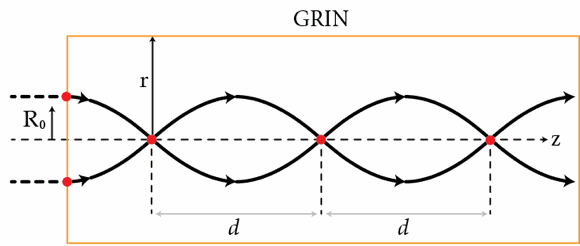# GRIN

Consider a cylindrical optical fiber with a core that has a graded index (GRIN) of refraction given by $n(r)=n_{0}\sqrt{1- (g r)^2}$ where $r$ is the distance to from the optical axis of the fiber. Show that this profile results in continual refocusing of paraxial rays as shown in the figure below. If $g=0.33~\mbox{mm}^{-1}$, determine the distance $d$ in millimeters between two consecutive focal points.×

Problem Loading...

Note Loading...

Set Loading...